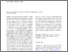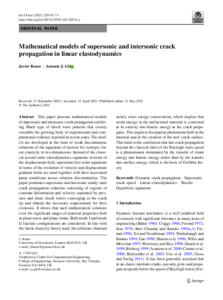Mathematical models of supersonic and intersonic crack propagation in linear elastodynamics

and (2021) Mathematical models of supersonic and intersonic crack propagation in linear elastodynamics. International Journal of Fracture, 229. pp. 55-75. ISSN 0376-9429 (Print), 1573-2673 (Online) (doi:https://doi.org/10.1007/s10704-021-00541-y)Preview
PDF (Publisher's PDF - Oen Access)
32734 BONET_Mathematical_Models_of_Crack_Propagation_(OA)_2021.pdf - Published Version

Abstract

This paper presents mathematical models of supersonic and intersonic crack propagation exhibiting Mach type of shock wave patterns that closely resemble the growing body of experimental and computational evidence reported in recent years. The models are developed in the form of weak discontinuous solutions of the equations of motion for isotropic linear elasticity in two dimensions. Instead of the classical second order elastodynamics equations in terms of the displacement field, equivalent first order equations in terms of the evolution of velocity and displacement gradient fields are used together with their associated jump conditions across solution discontinuities. The paper postulates supersonic and intersonic steady-state crack propagation solutions consisting of regions of constant deformation and velocity separated by pressure and shear shock waves converging at the crack tip and obtains the necessary requirements for their existence. It shows that such mathematical solutions exist for significant ranges of material properties both in plane stress and plane strain. Both mode I and mode II fracture configurations are considered. In line with the linear elasticity theory used, the solutions obtained satisfy exact energy conservation, which implies that strain energy in the unfractured material is converted in its entirety into kinetic energy as the crack propagates. This neglects dissipation phenomena both in the material and in the creation of the new crack surface. This leads to the conclusion that fast crack propagation beyond the classical limit of the Rayleigh wave speed is a phenomenon dominated by the transfer of strain energy into kinetic energy rather than by the transfer into surface energy, which is the basis of Griffiths theory.View Item